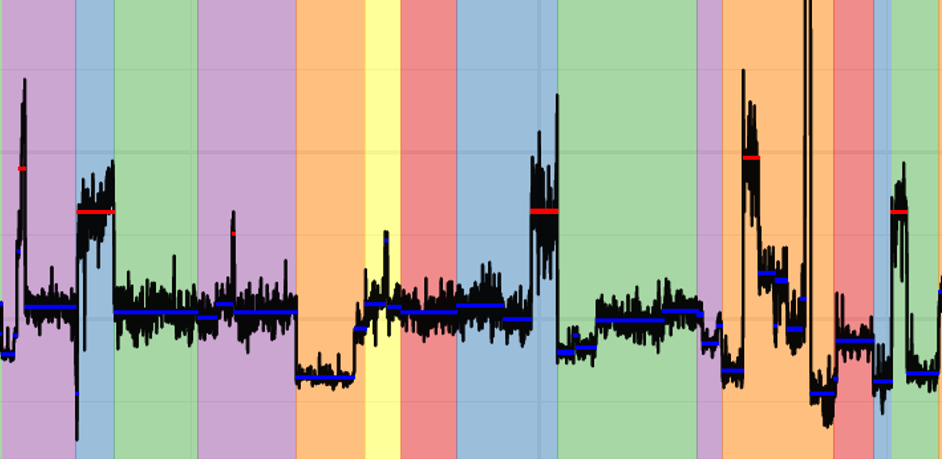# CNV detection II: Tumor CNV calling without matched normal

Ghost wrote a blog regarding tumor CNV calling with matched normal earlier. Here let's talk about what we can do when matched normal is not available. We still use targeted sequencing data where probe efficiency is the major problem.

Tumor CNV calling with panel of tumor

If we are processing a large number of tumor sample, say above 50 samples, we can use all 50 tumor sample themselves as reference. Of course, some pre-processing is needed beforehand.

1. For each tumor sample, normalize each amplicon depth against library size.

2. Some of the tumor samples probably contain CNVs somewhere. We would like to exclude these regions by defining outliers in boxplot.

3. For each amplicon, generate reference of normalized mean depth and standard deviation across samples.

Once we got our reference from panel of tumor, we can calculate z-scores between testing sample and reference distribution (defined by mean and std) for each amplicon. Next is the aggregation step where we take averaged z-scores per gene and calculate z-scores again against reference.

Below is an example output where x-axis is chromosome and y-axis is z-score with cutoff being 2.5:Note that the final z-score is calculated from averaged z-score of many amplicons within gene. The line segment in the figure indicates one or more genes, but amplicons.

Also, depending on the panel, each chromosome may not include all genes within each chromosome, but only the genes that being targeted. That is why we see disordered length of each chromosome.

CNV significance depends on amount of reads

In general, the success of calling CNV depends on the total amount of reads laying within the interval. If the sequencing depth is not high enough, we can always expand the window size. However, keep in mind that window size and detection resolution are trade-off.

From past experience, it seems that 0.25 mean coverage for each 50 kb window (0.25 * 50kb = 12.5kb per window) is good enough to call CNV on WGS data. As a role of thumb, this depth requirement can be extrapolated.